### SLOPE Function Examples in Excel, VBA, & Google Sheets

This Excel Tutorial demonstrates how to use the Excel SLOPE Function in Excel to calculate the slope.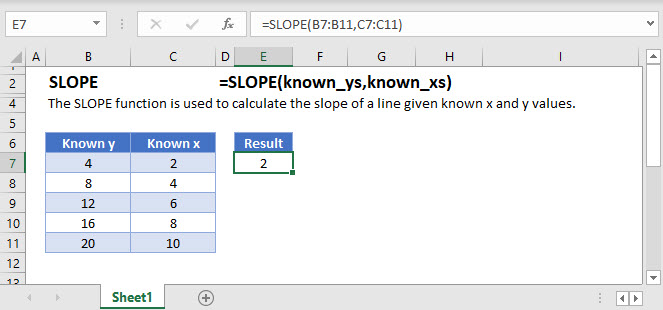## SLOPE Function Overview

The SLOPE Function Calculates the slope of a line generated by linear regression.

To use the SLOPE Excel Worksheet Function, select a cell and type: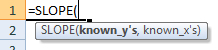(Notice how the formula inputs appear)

## SLOPE Function Syntax and inputs:

known_y’s – An array of known Y values.

known_x’s – An array of known X values.

AutoMacro - VBA Code Generator

## What is SLOPE?

The SLOPE function in Excel is used to calculate the slope of a line given known x and y values.

The SLOPE function can be used in conjunction with the INTERCEPT function to find the equation of a linear line,

Suppose I have a table of data with x and y values: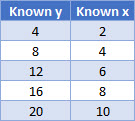The slope of a line is calculated by plotting the data and using the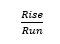method, demonstrated below: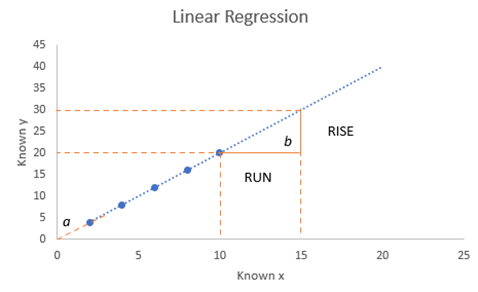Using the above chart, we can calculate the slope, b using the rise of the line divided by the run of the line.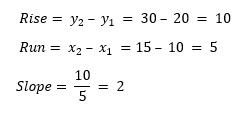Using the SLOPE function: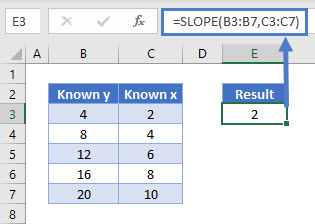## How to use SLOPE

The SLOPE function takes two arguments:

Where known_y’s and known_x’s refer to the x and y data in your data table.

Using our first example, the function is written as:

## Pairing SLOPE and INTERCEPT

To pair SLOPE and INTERCEPT, an equation can be written as:

## Interested in More Forecasting?

Pair SLOPE with INTERCEPT to see how to create the equation of a line and visit our other articles on Forecasting with Exponential Smoothing, TREND and LINEST functions.

## SLOPE function in Google Sheets

The SLOPE function works exactly the same in Google Sheets as in Excel.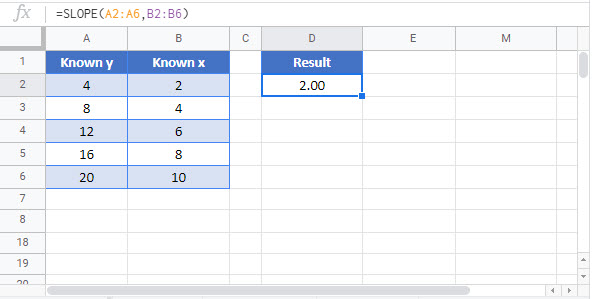## Additional Notes

The slop of the regression line is calculated as <<<<<<<<<<<>>>>>>>>>>>>>>

Known_y’s and Known_x’s must not be empty and must have the same number of data points.

## SLOPE Examples in VBA

You can also use the SLOPE function in VBA. Type:
`application.worksheetfunction.slope(known_ys,known_xs)`
For the function arguments (known_y’s, etc.), you can either enter them directly into the function, or define variables to use instead.

Return to the List of all Functions in Excel

## Excel Practice Worksheet

Practice Excel functions and formulas with our 100% free practice worksheets!

• Automatically Graded Exercises
• Learn Excel, Inside Excel!